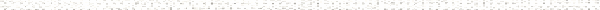A Precalculus Outline

 Chapter 10:  Foundations of TrigonometryObjective: Understanding radian measure and converting between degree and radian measure.Objective: Oriented Angles in Standard PositionObjective: Finding Coterminal Angles in DegreesObjective: Finding Coterminal Angles using Radian measureObjective: Linear and Angular VelocityObjective: Arc Length and Area of a SectorObjective: Types of Angles: Acute, Obtuse, etc.Objective: Complementary and Supplementary AnglesObjective: Circle Basics: radius, diameter, circumference, area and PiObjective: Defining Sine and Cosine using the Unit CircleObjective: Help Memorizing Values on the Unit CircleObjective: Reference AnglesObjective: Right Triangle TrigonometryObjective: Special Triangles (45-45-90 and 30-60-90)Objective: Using the Quotient, Reciprocal, and Pythagorean IdentitiesObjective: Verifying IdentitiesObjective: Right Triangle TrigonometryObjective: Using a calculator to approximate trigonometric valuesObjective: Solving Right TrianglesObjective: Applications of Right Triangle TrigonometryObjective: The Unit Circle: Cosine and Sine (Section 10.2)Objective: Simplifying radicalsObjective: Simplifying complex fractionsObjective: Sum and difference identitiesObjective: Double angle identitiesObjective: Power reducing identitiesObjective: Half angle identitiesObjective: The Unit Circle: Cosine and Sine (Section 10.2)Objective: The Six Circular Functions and Fundamental Identities (Sectioni 10.3)Objective: Simplifying radicalsObjective: Simplifying complex fractionsObjective: Animation of cosine and sine graphsObjective: Basic GraphsObjective: Transformations of the graphs of the Trigonometric FunctionsObjective: The Unit Circle: Cosine and Sine (Section 10.2)Objective: The Six Circular Functions and Fundamental Identities (Sectioni 10.3)Objective: Graphs of functions (Section 1.6)Objective: Transformations of graphs (Section 1.7)Objective: Inverse Circular FunctionsObjective: Inverse functionsObjective: The Unit Circle: Cosine and Sine (Section 10.2)Objective: The Six Circular Functions and Fundamental Identities (Section 10.3)Objective: Graphs of the Trigonometric Functions (Section 10.5)Objective: Solving basic equationsObjective: Solving equations which are quadratic in form using identitiesObjective: Solving equations using the double angle formulaObjective: A mix of more advanced techniquesObjective: The Six Circular Functions and Fundamental Identities (Section 10.3)Objective: Trigonometric Identities (Section 10.4)Objective: FactoringObjective: Sign diagrams